﻿ A Modified Model of the Universe Shows How Acceleration Changes Galaxy DynamicsPublications are Open
Access in this journal
Article Versions
Export Article
• Normal Style
• MLA Style
• APA Style
• Chicago Style
Research Article
Open Access Peer-reviewed

A Modified Model of the Universe Shows How Acceleration Changes Galaxy Dynamics

Jarl-Thure Eriksson
International Journal of Physics. 2018, 6(2), 38-46. DOI: 10.12691/ijp-6-2-3
Published online: April 11, 2018

Abstract

Based on the hypothesis that the combined matter and radiation energy of the universe is balanced by an equivalent amount of negative gravitational energy, a novel formulation of the Friedmann equation is examined. The hypothesis opens new avenues for studying the evolution of the universe. The expansion, initiated by the event of a vacuum fluctuation, is driven by the continuous occurrence of new positron-electron pairs. The current acceleration of expansion, gexp = 1,066·10-11 m/s2, emerges as a result of the study. It is mathematically shown that gexp causes a cosmic Coriolis effect responsible for the rotary velocity deviations in the galaxies. The effect eliminates the need for dark matter. The MOND theory is explained on a purely physical basis.

1. Introduction

During the last couple of decades conflicting ideas about the evolving universe have put traditional cosmological models to the test. The accelerating expansion of the universe is one significant new discovery, cf. Perlmutter 1. More controversial issues are the lack of candidates for dark matter and discrepancies in the gravitational fields of galaxies. The origin of dark energy is still a mystery. Alan Guth has been the proponent of the idea, that all the energy in the universe sum up to zero, i.e. matter and radiation are balanced by the negative potential energy provided by gravitation 2.

In 1973 Edward P. Tryon suggested that the universe started from an electron-positron vacuum fluctuation 3, an attractive idea that has been elaborated in many papers ever since. Electron-positron vacuum fluctuations are known for a fact, however, pairs are very short-living due to the Heisenberg uncertainty principle. The electron and its antiparticle are interesting because of their stable nature and as beholders of the electric charge, the origin of electromagnetism. Recently (2014) H. Dongshan, G. Dongfeng and C. Qing-yu published a mathematical proof of how spontaneous fluctuations create a bubble, which is able to sustain itself due to the fast expansion 4.

An implication of the zero energy hypothesis is that the universe is growing due to a continuos addition of new matter. As a logical consequence, there should be a connection between the energy increase and the metric expansion, whereby existing models of an expanding universe based on solutions to the classic Friedmann equation are erroneous. In this study the Friedmann-Lamaître-Robertson-Walker metric will be reformulated and the consequences analyzed.

In the latter part of the paper an equation is derived to determine the relation between the cosmic age and the proper distance, i.e. the light cone space-time diagram. The profile equals similar diagrams, but the best fit requires that the Hubble constant H0 is given the right choice, here H0 = 68,24·103 m/s/Mpc. This is actually the only adjustment needed to complete the present model, which is called the Continuously Breeding Universe model or CBU for short.

The dynamics of galaxies are studied from the rotational velocity point of view. It is found that a factor depending on the expansion acceleration gexp must be added to the Newtonian acceleration in order to provide rotational velocities consistent with expected real values. Furthermore gexp “infects” observed velocities by influencing the scaling of the measurements. S. McGaugh et al. 5, 6 and G. M. Eadie et al. 7 have recently published results which strongly support these interpretations.

2. Basic Assumptions

In the early 20th century two evident cosmological coincidences intrigued physicist. As a result new ideas concerning the connection between gravitation and particle physics emerged. Paul Dirac presented his large number hypothesis (LNH) in 1937 8. Before him, however, Hermann Weyl, 9, and Arthur Eddington 10, had speculated over the interpretation of "the most mysterious, unexplained phenomena in cosmology" 11. One specific large number N is obtained from the ratio between the radius of the universe ru and the radius of the proton rp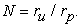(1)

According to one of the coincidences the total matter content, mass Mu, is proportional to the number N squared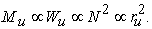(2)

This may be considered as the first coincidence.

The present investigation is based on three assumptions. The first assumption claims that the energy of the universe, in accordance with eq. (2), is proportional to the surface of a sphere having a radius ru, at a specific time t. Definition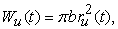(3)

where Wu(t) is the total (positive) energy of the universe. The constant b is derived from known constants of Nature, ru is the virtual over all radius of the universe. The deliberately chosen definition of eq. (3) will be obvious at a later stage. We will find that the results of this assumption is in good agreement with present estimates of the energy content of the universe.

The second assumption states that the universe was initiated by a positron-electron fluctuation. The expansion of the universe is intimately coupled to a continuous succession of fluctuations. In 1974 Dirac extended the LN hypothesis by introducing the possibility of "additive creation", i.e. the spontaneous addition of matter all through the universe 13. Only a fraction of positron-electron pairs “stay alive”, but that fraction is responsible for the accumulated increase of the metric space.

The third assumption requires that we define the radius r of the observable universe. We assume that the diameter of the observable universe equals the radius of an outer virtual boundary of the universe, r = ru/2. This is supported by recent reports, cf. P. Bielewicz et al. 14, ru = 8,609·1026 m (here: 2r0 = 8,412·1026 m, where r0 is the present value of r).

The third assumption is related to the hypothesis stated by Alan Guth 2, and further by Lawrence Krauss 15, saying that the energy sum is zero. If a spontaneous creation of matter, e.g. m = 2me, happens at the ”virtual edge” (ru) of the universe, then from basic physics we get a relation between the gravitational constant G, the radius and the total mass Mu (including the equivalent mass of the radiation energy)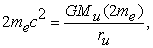(4a)

or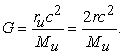(4b)

For any observer there will be an ”edge” somewhere else in the universe, which leads us to the assumption that the fluctuations of positron-electron pairs happen spontaneously and isotropically at any point in space. Notice that eq. (4b) does not depend on me.

Eq. (4b) was identified by Arthur Eddington and much earlier by Ernst Mach as a significant cosmological relation having a special bearing on the so called Mach's principle, 11, 16. In 1952 Dennis Sciama published a paper "On the origin of inertia" 17, wherein he referred to Mach's work and concluded that the relation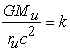(5)

emerges from the theory. k is close to 1. Eq. (4b) may be considered as the second coincidence recognized by the physicists. By substituting Mu = Wu/c2 = 4πbr2/c2 into eq. (4b) we obtain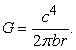(6)

The gravitational constant G is dependent on radius r, and time t, a much debated issue. Later we shall see that the change over time is below present resolution limits.

3. The Initial Event

The origin of the first positron-electron fluctuation will remain a question not answered here. But once it happened it also gave birth to a metric space and a vacuum within the space. We have to be careful not to give vacuum the same mysterious mission as the ether had a century ago. However, in combination with the metrics, it will provide change, that is time, by sparkling new e+e- pairs.

At the initial event the positron-electron pair creates a structure, wherein the particles are opposite to each other on the comoving surface of the initial universe, radius ri. The distance between the particles is πri. After a while more pairs are formed and some of them annihilate to give birth to the first bursts of EM-radiation. At the initial event the energy equation of the system is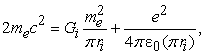(7)

where me and e are the electron mass and charge respectively. From eq. (4b) we have the gravitational parameter Gi = 2ric2/2me. The comoving radius of the initial universe is obtained from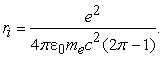(8)

The value is ri = 0,533381.10-15 m.

Based upon the information above it is possible to define a modified Dirac ”large number” ND’= (WG+We)/WG, where WG and We are the energies of the gravitational and electric fields respectively. It appears that ND’ at the initial event is 2π, a beautiful result.

We use the initial event to determine the energy constant b. Based on the definition in eq. (3) we write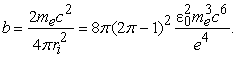(9)

The value is, b = 0,458009.1017 J/m2.

From eq. (6) we are now able to determine the radius of the observable universe today, r0. By using the value of G0 = 6,67389·10-11 Nm2/kg2 the radius is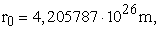which is 1,9 % smaller than the latest estimate, 4,289·1026 m 18. One explanation might be that G0 as measured in the solar system, is influenced by discrepancies in the local curvature, such as the gravitational fields of the sun, nearby clusters and the location in the Milky Way. It is noteworthy that measurements on G0 varies in the 4th decimal.

By inserting b from eq. (9) into eq. (6) we obtain an equation for G consisting of known physical constants times the ratio 1/r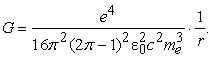(10)

Time dependence of r and local deviations in the curvature of space invalidate the use of Newton’s law of gravitation on a large cosmological scale.

4. Equation of Expansion

Albert Einstein compared the dynamic equations of general relativity with the Eulerian hydrodynamical equations for an incompressible fluid, whereby he introduced the concepts of the density ρ and the pressure p into his theory 19. Alexander Friedmann went further on this idea and derived the equations of the time derivative and the acceleration of a scale factor a = r/r0 describing the expansion of an homogenous, isotropic universe 20. We focus on the acceleration equation in accordance with the Friedmann-Robertson-Walker metric, cf. 21,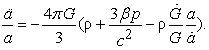(11)

The equation takes into account the time change of G. Both the mass and the radiation energy are included in the density ρ.

We multiply the pressure by a new parameter β ("bang-factor") in order to calibrate the pattern of the observed expansion. The parameter takes into account the extra pressure provided by the continuous addition of new matter. This is partly in conflict with Einstein's cosmological constant λ 22, which has no obvious physical explanation. However, when knowing β we can estimate λ and thereby provide a background to the cosmological constant

From the relations W = πbru2 and dW = 2πbru·dru = -pdV, we derive p = - b/2ru = - b/4aro. Further from eqs. (3) and (6) we have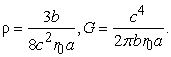Ġ is obtained by derivation of G. These quantities as functions of the scale factor a are inserted into eq. (11).

The final equation is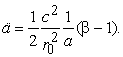(12)

Compared to the traditional Friedmann-Robertson-Walker expression eq. (12) leads to a different description of the evolution of the universe. Interestingly the assumptions of Section 2 leads to this simple equation, which shows that for β = 1 the acceleration becomes zero, the stationary case. Expansion requires that β > 1.

As a first step in solving eq. (12) we write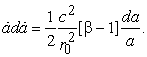(13)

By integration we obtain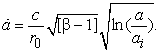(14)

Here ai = ri/r0 = 1,2682·10-42.

Next we use the substitution u = ln(a/ai) and arrive at the following integral equation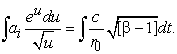(15)

The solution to the left side is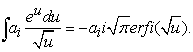(16)

The real part solution of the error function is obtained by using the Dawson integral function D+(√ln a/ai). We can now write the equation for the expansion time t, i.e. the cosmic age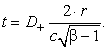(17)

Notice that ar0 = r has been substituted into the equation.

The determination of the parameter β will be the only necessary adjustment of the present model with current, generally accepted measurements. We solve β from eq. (14) presuming that the Hubble constant is H0 = 68,24·103 m/s/Mpc for a = 1 (H0 from section 6). The result is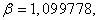which is slightly greater than 1 indicating the expansion.

The time needed for the universe to reach its present size appears to be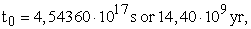or about 3,9 % larger than recently estimated.

The scale factor a as a function of normalized time τ = t/t0 is shown in Figure 1. Also shown are some of the supernova data presented by Saul Perlmutter 1. The function a = f(τ) is very close to a straight line. The comoving radius can with good accuracy be estimated from the curve fit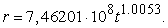(18)

The acceleration of the present universe expansion is obtained from eq. (12)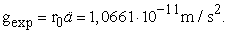From the same equation we can extract gu = c2/2r0a (g was chosen as the common symbol of acceleration to avoid a mix-up with the scale factor a), which for a = 1 takes the value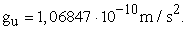Mordehai Milgrom, the proponent of the MOND theory, recognizes a connection between the MOND acceleration 1,2·10-10 m/s2 and c2/Ru, where Ru is the “radius of the Universe”, 23. Here Ru = 2r0.

The change of the gravitational parameter G over time can roughly be estimated by substituting eq. (18) into eq. (6) and then by determining the time derivative. As a result, the present rate of change is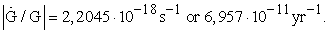Based on observations of the pulsating white dwarf star G 117-B 15A, Benvenuto et al. 24 have set the resolution limit to 2,5·10-10 yr-1. Observing the same white dwarf Biesiada et al. 25 suggest a limit of 4,1·10-10 yr-1. The calculated change is clearly below the estimated resolution limits. It’s interesting, though, that the limits and the model value are in the same range of magnitude.

• Figure 1. The scale factor as a function of time obtained as a semi-analytical solution to the modified Friedmann equation. The math requires the numerical values of the Dawson integral

5. Number of Positron-electron Fluctuations

The expansion is bonded to a mathematical pattern, which means that there must be a mechanism creating a steady delivery of new positron-electron pairs. This mechanism depends on the radius r, but is not influenced by the temperature nor the chaotic events of stellar collisions or nuclear reactions in stars. It suggests a still unknown law of physics, which may open new insight in the interplay between the metric space and quantum phenomena.

We now have the tools to illuminate the first moments of the emerging universe. By definition the total (positive) energy of the universe is Wu = 4πbr2 = Ne+e-·2mec2, where Ne+e- is the number of fluctuations needed for said energy Wu. Combining this with the first part of eq. (9) we obtain a useful relation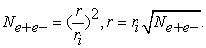(19)

Given Ne+e- we determine r and further from eq. (17) the time steps immediately following the initial event. The results are presented in Figure 2. The scale of time is 10-23 s and of the radius of the comoving sphere 10-15 m. The first step (r1= ri = 0,533256·10-15 m) is of the same order of magnitude as the charge radius of the proton, 0,84087·10-15 m 26.

A surprising result appeared, when the matter density of the whole universe was approximated. Recent NASA estimates of the density of ordinary matter is ρ = 4,36... 4,55·10-28 kg/m3, 27.

In the present study the total (positive) energy content of the universe is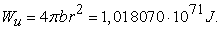(20)

Given the volume Vu = (4π/3)·ru3 = (32π/3)·r3 = 2,49299·1081 m3, the matter density is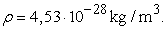The good agreement with NASA figures indicates a strong argument in favor of the hypothesis Wu ∝ r2.

• Figure 2. Universe size development after the initial event. Ne+e- is the number of positron-electron fluctuations needed for the specific state

6. The Cosmic Age to Proper Distance Diagram

The cosmic age to proper distance (light cone) diagram is an important tool in determining the history of the evolving universe. The Hubble constant H0 is determined by adjusting the maximum proper distance to a computed profile based on the Planck 2013 data. The result will establish the expansion parameter β.

We define the radius of the frontier of the expanding universe as rF = r/π, rF0 = r0/π for the present universe. From eq. (14) we obtain the velocity of the frontier expansion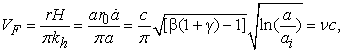(21)

where the Hubble constant H is in m/s/Mpc, H = H0 at a = 1, kh = 3,08567·1022 m/Mpc and ν is the ratio of the velocity VF to the speed of light. According to the present (CBU) model ν is smaller than 1, but will approach 1 with increasing time.

The geometry required for the calculation of the proper distance is seen in Figure 3. Our task is to determine the radius x as a function of the cosmic age tx. The perimeter of a circle of radius x represents the proper distance dp at t = tx.

• Figure 3. The frontier of the expanding universe and the geometry for the determination of the proper distance

We define a time factor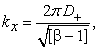(22)

such that tx = kxrF/c; t0 = k0rF0/c. (D+ is the Dawson integral function as introduced earlier.)

Based on the geometry in Figure 3 we deduce the following equation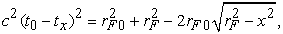(23)

wherefrom x is solved. We introduce the normalized quantities: κ = x/rF0; a = rF/rF0 = scale factor a. κ is obtained from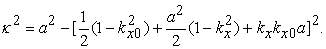(24)

The proper distance dp is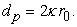(25)

The relation between the cosmic age and the proper distance is presented in Figure 4. The computed results are compared to the curve originally published by Davies and Lineweaver 28, however updated according to Planck 2013 satellite data, as implemented by N. Crichton in 2015 29. The only adjustment required was to obtain equal maximum proper distances dpmax= 5,89 Gly. As seen from Figure 4 the forms are almost identical. The adjustment resulted in the following key parameters: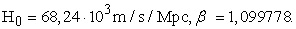The present velocity of the expansion frontier is 0,9876·c, i.e. 2,9619·108 m/s.

We can estimate the red-shift in the region z = 0...1 from the commonly known equation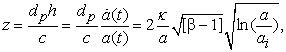(26)

where h = rH/kh. The red-shifts are indicated in Figure 4. The resemblance with Ref. 29 is fairly good, which supports the validity of eqs. (24) and (26). Eq. (26) does not consider the influence of the change in G, which should move the z points towards the present time.

In Figure 5 the angular diameter distance is compared to the Standard Model (ΛCDM) presented by Bonamente et al. 30. Also here the conformity is very good. It is noteworthy that the CBU model only contains one adjusted parameter, β, while the Standard Model is a statistical composition containing at least 6 adjustable parameters.

• Figure 4. The light cone diagram showing the cosmic age as a function of the proper distance. The “Planck” case is based on Ref. 
• Figure 5. The angular diameter distance as a function of the red-shift. The background data by Bonamente et al. Ref. 

7. The Cosmic Coriolis Force, an Alternative to Dark Matter

According to the web-site dictionary.com a fictitious force is “any force that is postulated to account for apparent deviations from Newton’s laws of motion appearing in an accelerated reference system.” If the expansion of the universe is accelerating as proposed by Perlmutter 1 and postulated here, this will have a fundamental impact on the dynamics of galaxies and stars. In evidence we shall derive an equation, which separates the “true” acceleration gtru from acceleration components measured by an outside observer.

Let O be the coordinate system of the observer, Figure 6. The dynamics of an object at P in the coordinate system A are under investigation. We define the position vectors as follows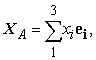(27)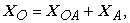(28)

where ei is the unit vector.

• Figure 6. An observer in system O is measuring the dynamics of a body at P in the coordinate system of A

The velocity measured by the observer is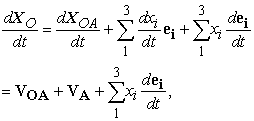(29)

where VOA is the velocity difference between the systems, VA the velocity of P in A. The last term represents the rate of expansion. The acceleration of P measured by the observer is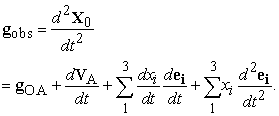(30)

For a completely outside observer the acceleration difference gOA equals the expansion acceleration gexp. However, if O belongs to the same galaxy system, gOA is zero.

We are especially interested in dVA/dt, which represents the “true” (in the reference frame of A) acceleration of the body at P. By derivation of the middle term VA in eq. (29) we obtain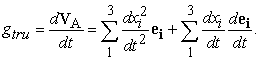(31)

The first term in the latter part of eq. (31) represents the Newtonian acceleration. The second term is due to the scale unit change, which in turn is a result of the expansion. This is referred to as a fictitious force, which is analog to the Coriolis force in rotating air and water flows along the surface of the Earth. Here the effect is called the Cosmic Coriolis force. As in the case of the ordinary Coriolis the term containing the product of dxi/dt and dei/dt occurs twice, once in the “true” acceleration and a second time in the observers reference frame. The term is called gkic and will have an important role in the further investigation.

For simplicity reasons we presume that A is a 2-dimensional cylindrical coordinate system, the x3-coordinate is set to zero, mimicking the thin disk configuration of galaxies. The equations of transformation between the Cartesian and the cylindrical system are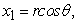(32a)(32b)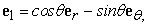(33a)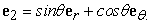(33b)

After the variables and unit vectors have been inserted into equations (30) and (31) and all necessary mathematical operations have been performed, the equations take the forms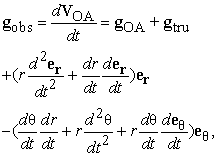(34)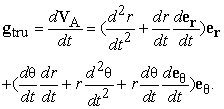(35)

When gtru is inserted in gobs, the tangential eθ components exclude each other, the observer will only register the radial components (assuming that gOA is zero).

In our search for an explanation to the deviation in the rotational velocities of stars we concentrate on the radial component of gtru. We denote

gN = d2r/dt2, the Newtonian gravitational acceleration in radial direction,

gexp = d2er/dt2, acceleration of the universe expansion, dr/dt = Vr and der/dt = Vexp/r.

We further denote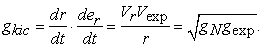(36)

The final equations of acceleration are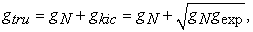(37)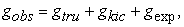(38)

The velocity of an orbiting object is obtained by applying the familiar law of the centripetal force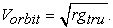(39)

In Figure 7 observed accelerations from different sources are compared with the calculated curves based on equations (37) and (38). A similar diagram has been used by McGaugh et al., 5, 6. It appears to be very instructive in the evaluation of different interpretations.

• Figure 7. The relation between observed/true accelerations and the Newtonian acceleration. The full lines represent the present theory, CBU, dashed curves indicate (i) the observed statistical result of McGaugh et al., [5,6], and (ii) an acceleration reconstruction of the Eadie et al., , dark matter halo. The dotted curve is a calculated version of the MOND acceleration

The results of McGaugh et al. show that the observed acceleration for decreasing gN approaches a constant minimum value ĝ = 0,92·10-11 m/s2. It seems obvious that ĝ is identical with the acceleration of the universe expansion, gexp, as hypothesized here. Observations collected by McGaugh et al. provide mixed messages, some objects, the Milky Way satellite galaxies, say, require the additional gkic, whilst Andromeda (M31) satellites do not.

Interestingly the MOND curve almost exactly follows the Coriolis scheme: gMOND = gN + 2gkic, however lacking the term gexp. There is a separate discussion of the results of Eadie et al. in the next section.

8. The Rotational Velocity of the Milky Way

Deviations from the Keplerian scheme in the rotational velocities of galactic bodies were first reported by Vera Rubin 31. The existence of a hitherto unobservable form of matter, dark matter, was a logical solution to the discrepancy. In the previous section, however, we have seen that an alternative approach is possible. In order to verify this hypothesis, we start by looking for a dynamic model of the Milky Way. For simplicity reasons we assume that the galaxy consists of a spherical bulge and a thin disk, Figure 8. The disk is divided into three regions each having a constant surface mass density. Seven points have been selected for the estimation of the gravitational potential ϕ. The masses are:

MMW = 2,3·1041 kg, total mass of ordinary matter in the Milky Way,

mbulge = 0,29·1041 kg.

The masses and the surface densities, σ, have been selected as the result of an adjustment process, the goal of which has been to find the best possible accuracy of the velocity distribution. Feng and Gallo have suggested MMW = 2,19·1041 kg 32. The mass distribution in their paper follows the same trend as chosen here.

The gravitational potential has been determined by utilizing the equation derived by Lass and Blizen 33. The potential is obtained from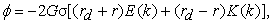(40)

where k = 2√(rdr)/(rd+r), rd is the outer radius of the disk, K(k) and E(k) are the elliptic integrals of 1st and 2nd degree respectively. Eq. (40) is valid both inside and outside the disk. The potential of an annular disk is obtained by subtracting the potential due to the equivalent disk of the hole.

Once we have carried out the computation of the complete potential ϕ(r) at every point, the Newtonian acceleration is obtained from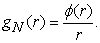(41)
• Figure 8. The principle geometric model of the Milky Way

The observed and the “true” velocities are obtained from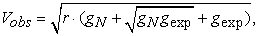(42)(43)

The velocities are compared with two different sets of measurements in Figure 9. The agreement between computed and measured values is good. As expected the “true” velocity drops monotonically with increasing radius. For the velocity curves Vobs to fit measurements they must not contain the second gkic term, cf. eq. (38). The obvious reason is that the observer rotate in the same reference frame as the object of measurement.

In January 2017 G. M. Eadie, A. Springfield and W. E. Harris (ESH) published a comprehensive analysis of the mass distribution of the Milky Way 7. Utilizing a hierarchical Bayesian approach they have developed a distribution function f(ε,L) to model the galaxy and the kinematic data from globular clusters in order to trace the gravitational potential of the MW. Even if the goal of Eadie et al. is the mapping of the dark matter halo, the results can be interpreted as evidence of the Cosmic Coriolis hypothesis.

Based on the mass profile MESH of Figure 4 in Ref. 7 we reconstruct gkic from the equation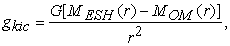(44)

where MOM(r) is the cumulative ordinary matter profile computable from eq. (41) (MOM = rϕ(r)/G). We then obtain gtru as a function of gN from eq. (37). The curve gtru (ESH) is seen in Figure 7. It is noteworthy that the MESH profile does not contain the extra Coriolis components gkic and gexp.

Conversely we can use the model of Figure 8 to reconstruct a virtual dark matter halo. This is obtained from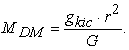(45)
• Figure 9a. The rotational velocity distribution of the Milky Way. M.J. Raid et al. 
• Figure 9b. The rotational velocity distribution of the Milky Way. Lamost 

The total mass, MMW = MOM+ MDM, is seen in Figure 10 together with MESH. The similarity is surprisingly good, considering that the data come from two completely different approaches. Minor discrepancies are explained by a smaller bulge mass and a denser mass profile of the present model. The similarity emphasizes the credibility of the total mass of ordinary matter in the Milky Way, MMW = 2,3·1041 kg.

9. Summary and Conclusions

The present study emphasizes the validity of Einstein’s General theory of relativity. It even extends the theory by demonstrating that the metric space is a measure of the total (positive) energy content of the universe.

The algorithm leading to the proper length diagram of Figure 4 provides a strong argument in favor of the CBU theory of the present paper. A pure mathematical procedure, eqs. (21)...(25), results in a profile perfectly fitting a computed profile based on Planck 2013 satellite data. The Hubble constant takes the value 68,24 km/s/Mpc, which is very close to the most recent estimates. Another argument is obvious from Figure 1, the almost linear nature of expansion fits the supernova data published by Perlmutter emphasizing the inadequacy of the classical Friedmann solution.

• Figure 10. Mass profile of the Milky Way. The full line is a reconstruction of the mass of ordinary and virtual dark matter based on the model in Figure 8. The dashed line is from Figure 4 in Ref.  and is based on a hierarchical method and 143 Globular Clusters

It is shown that the gravitational constant G is inversely proportional to the radius of the observable universe. Local deviations in the radius makes it impossible to define a universal constant G even for a particular moment in time. This partly explains why measurements of G in our solar system varies in the 4th decimal. In the early universe G is much bigger than now, which means that gravitation has influenced the redshift stronger than hitherto estimated. As a consequence early galaxies are younger than generally assessed.

The expansion factor β has been considered as a time invariant parameter. This may be true on a larger time scale, e.g. τ = 0,1...1, but not in the early phase of expansion.

The derivation of the Cosmic Coriolis effect is a decisive accomplishment of the present paper. The theory introduces additional factors to the gravitational acceleration. One factor, gkic, will physically change the kinematics of celestial bodies. Another factor, gexp, will influence the measurements made by a remote observer. In a wider intergalactic perspective a rigorous reformulation of the dynamic equations is required.

The analysis of Section 8 is concerned with the Milky Way, wherein the time differences between distant locations are small on a universal time scale. The hypothesis that G depends on the scale factor and on time was not taken into account in the analysis, the influence being seen only in the 4th decimal. However, extending the analysis to other galaxies the time dependence of G must be included, a fact that will considerably increase complexity.

The study raises several principal questions. Firstly, what physical law lies behind the extremely robust connection between positron-electron fluctuations and the expansion of the metric space? Secondly, what is the chain of processes transforming electrons and positrons of “the cold open space” into protons, neutrons and atoms? Does micro/mini black holes create matter which at a critical transition point converts into a variety of baryonic particles?

The origin of the CMB is also unclear. It is proposed that a certain ratio of the positron-electron pairs annihilated at the very beginning of the universe, thereby providing the energy equivalent with the background radiation. An isotropic annihilation process would explain both the ideal black body distribution and the uniformity over the sky.

The results put forward by the present study are in surprisingly good conformity with generally accepted data of today, such as universe size, age, matter density and the acceleration of expansion.

References

  Perlmutter, S. Supernovae, Dark Energy, and the Accelerating Universe, Physics Today, April 2003. In article View Article  Guth, A. The Inflationary Universe: The quest for a new theory of cosmic origins, Perseus Books, 1997. In article  Tryon, E. P. Is the universe a vacuum fluctuation?. Nature 247, pp. 396-397, 1973. In article View Article  Dongshan, H., Dongfeng, G., Qing-yu, C., Spontaneous creation of the universe from nothing. arXiv:1404.1207v1, 2014. In article View Article  McGaugh, S. S., Lelli, F., The radial acceleration relation in rotationally supported galaxies, arXiv:1609.05917v, 2016. In article  Lelli, F., McGaugh, S. S., Schombert, J. M., Pawlowski, M. S., One law to rule them all: The radial acceleration relation of galaxies, arXiv:1610.08981v1, 2016. In article View Article  Eadie, M. G., Springford, A., Harris, W. E., Bayesian mass estimates of the Milky Way, arXiv:1609.06304v3, 2016. In article  Dirac, P. A. M., The cosmological constants, Nature 139, 323, 1937. In article View Article  Weyl, H., Eine neue Erweiterung der Relativitätstheorie, Ann. Phys. 364, 101, 1919. In article View Article  Eddington, A., Preliminary note on the masses of the electron, the proton and the universe, Proc. Cam. Phil. Soc., 27, 1931. In article View Article  Unzicker, A., The relativity of inertia and reality of nothing, arXiv:0708.3518v5, 2011. In article  Ray, S., Mukhopadhyay, U., Ghosh, P. P., Large number hypothesis: A review, arXiv: 0705.1836v1, 2007. In article View Article  Dirac, P. A. M., Cosmological models and the large numbers hypothesis, Proc. R. Soc. London A 338, pp. 439-446, 1974. In article View Article  Bielewicz, P., Banday, A. J., Gorski, K. M., Constraints on the topology of the universe derived from the 7-year WMAP CMB data and prospects of constraining the topology using CMB polarization maps, arXiv:1303.4004v1. In article  Krauss, L., Universe from nothing, Simon & Schuster, NY, 2012. In article  Mach, E., History and root of the principle of the conservation of energy, The Science of Mechanics 6th ed. 1904. In article View Article  Sciama, D. W., On the origin of inertia, Monthly Notices of the Royal Astronomical Society 113, 34-42, 1952. In article View Article  Halpern, P., Tomasello, N., Size of the observable universe, Advances in Astrophysics Vol. 1. No. 3, 135-137, 2016. In article View Article  Einstein, A., Die Grundlage der allgemeine Relativitätstheorie. Annalen der Physik, 49, 769-822, 1916. In article View Article  Friedmann, A., Über die Krümmung des Raumes. Zeitschrift für Physik, Vol. 10, 377-386, 1922. In article View Article  Ohio State University, Astronomy 5682: Introduction to Cosmology, Spring 2009. In article  Einstein, A., Kosmologische Betrachtungen zur allgemeinen Relativitätstheorie. Sitzungsberichte der Preussischen Akad. d. Wissenschaften, 1917. In article View Article  Milgrom, M., MOND theory, arXiv:1404.7661v2, 2014. In article View Article  Benvenuto, O. G., Garcia-Berro, E., Isern, J., An upper limit to the secular variation of the gravitational constant from white dwarf stars, Phys.Rev. 69, 2004. In article View Article  Biesiada, M., Malec, B., A new white dwarf constraint on the rate of change of the gravitational constant, Monthly Notices of the Royal Astronomical Society, 350, 644-648, 2004. In article View Article  Antognini, A. et al., Proton structure from the measurement of 2s-2p transition frequencies of muonic hydrogen, Science Vol. 339, 417-20, 2013. In article View Article  PubMed  NASA, Aeronautics and Space Administration, Universe 101, 2014. In article  Davies, T., Lineweaver, C. H ., Expanding confusion: common misconceptions of cosmological horizons and the superluminal expansion of the universe, arXiv: astro-ph/0310808v2, 2003. In article View Article  Crighton, N., Make a plot with both redshift and universe age axes using astropy. cosmology. Ipython.display. Cosmological Calculations, 2015. In article  Bonamente, M., Joy, M. K., LaRoque, S. J., Carlstrom, J. E., Reese, E. D., Dawson, K. S., Determination of the cosmic distance scale from Sunyaev-Zeel'dovich effect and Chandra x-ray measurements of high redshift galaxy clusters, arXiv:astro-ph/0512349v2, 2006. In article View Article  Rubin, V. C., Thonnard, N., Ford, W. K., Extended rotation curves of high-luminosity spiral galaxies, Astrophysical Journal Letters 225, L107, 1978. In article View Article  Feng, J. Q., Gallo, C. F., Galactic rotation described with bulge+disk gravitational models, arXiv:1007.3778v3, 2011. In article View Article  Lass, H., Blitzer, L., The gravitational potential due to uniform disks and rings. Celestial Mechanics 30, 225-228, 1982. In article View Article  Reid, M. J., Menten, K. M., Brunthaler, A., Zeng, X. W., Dame, T. M., Xu, Y., Wu, Y., Zhang, B., Sanna, A., Sato, M., Hachisuka, K., Choi, Y. K., Immer, K., Moscadelli, L., Rygl, K. L. J., Bartkiewicz, A., Trigonometric parallaxes of high mass star forming regions: the structure and kinematics of the Milky Way, arXiv:1401.5377v3, 2014. In article View Article  LAMOST Survey, The Milky Way’s rotation curve out to 100 kpc and its constraint on the Galactic mass distribution, Press release November 18, 2016. In article View ArticleThis work is licensed under a Creative Commons Attribution 4.0 International License. To view a copy of this license, visit http://creativecommons.org/licenses/by/4.0/